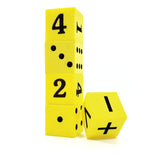•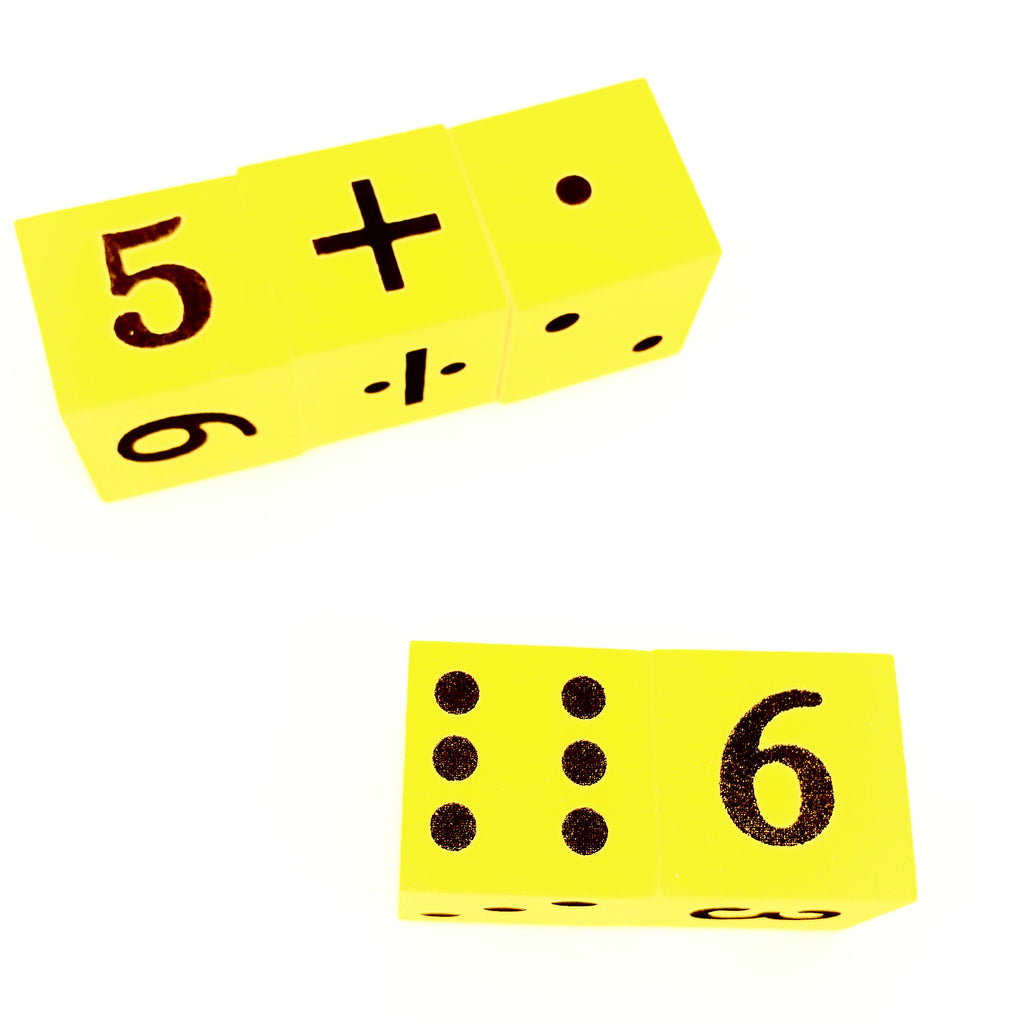•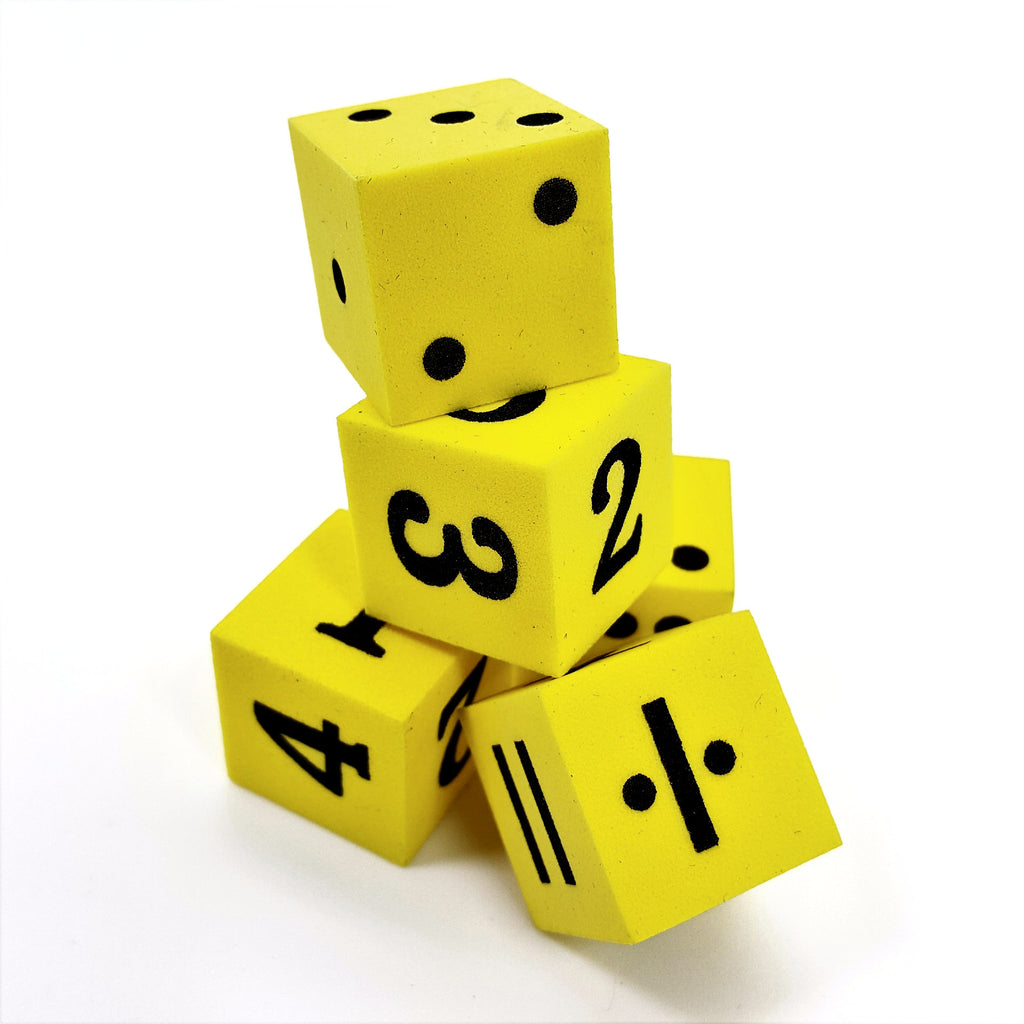•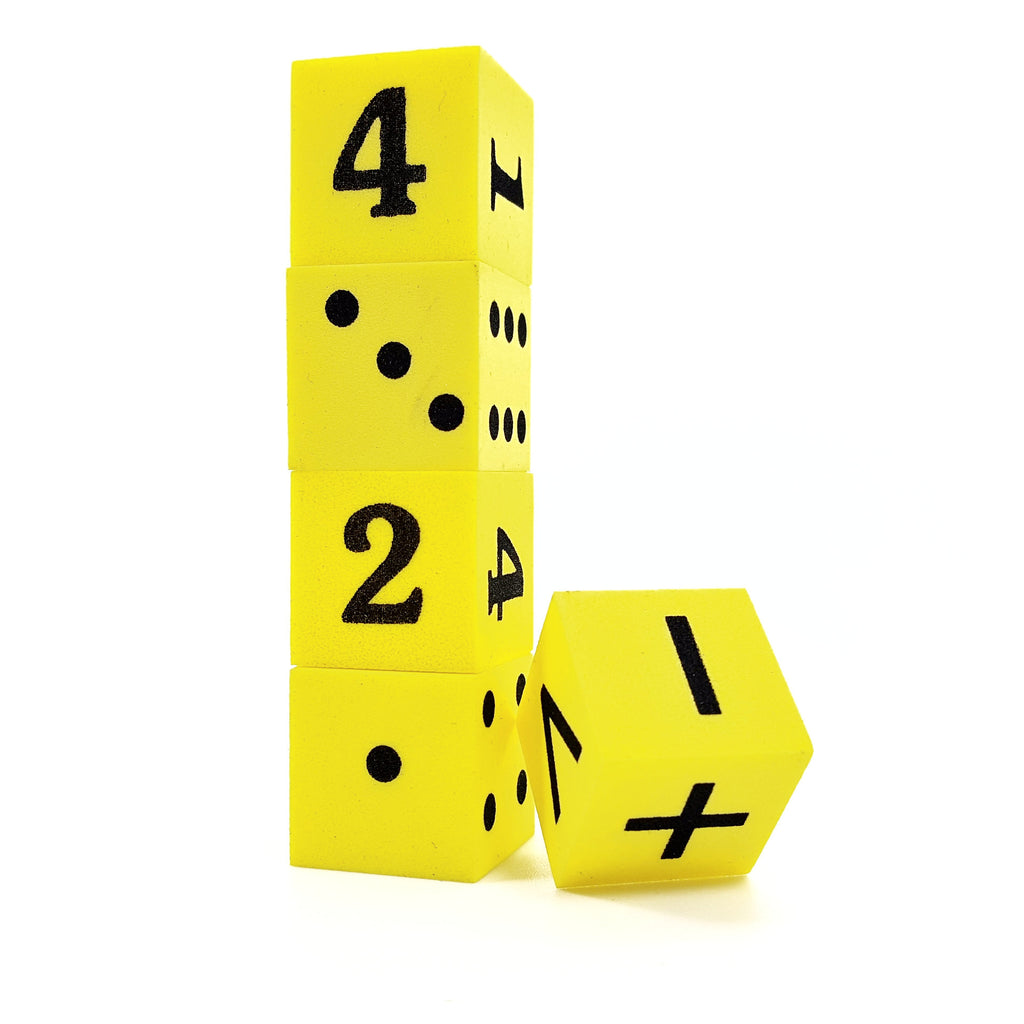•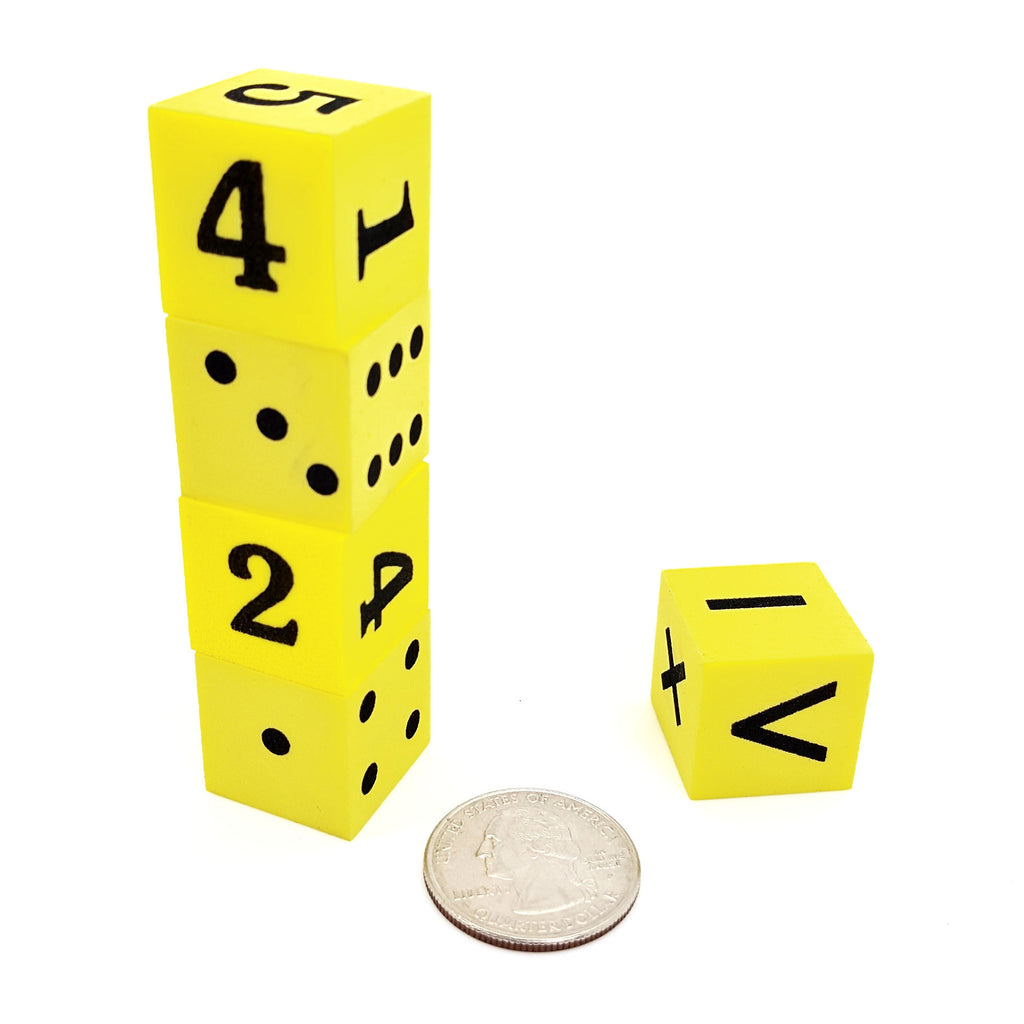•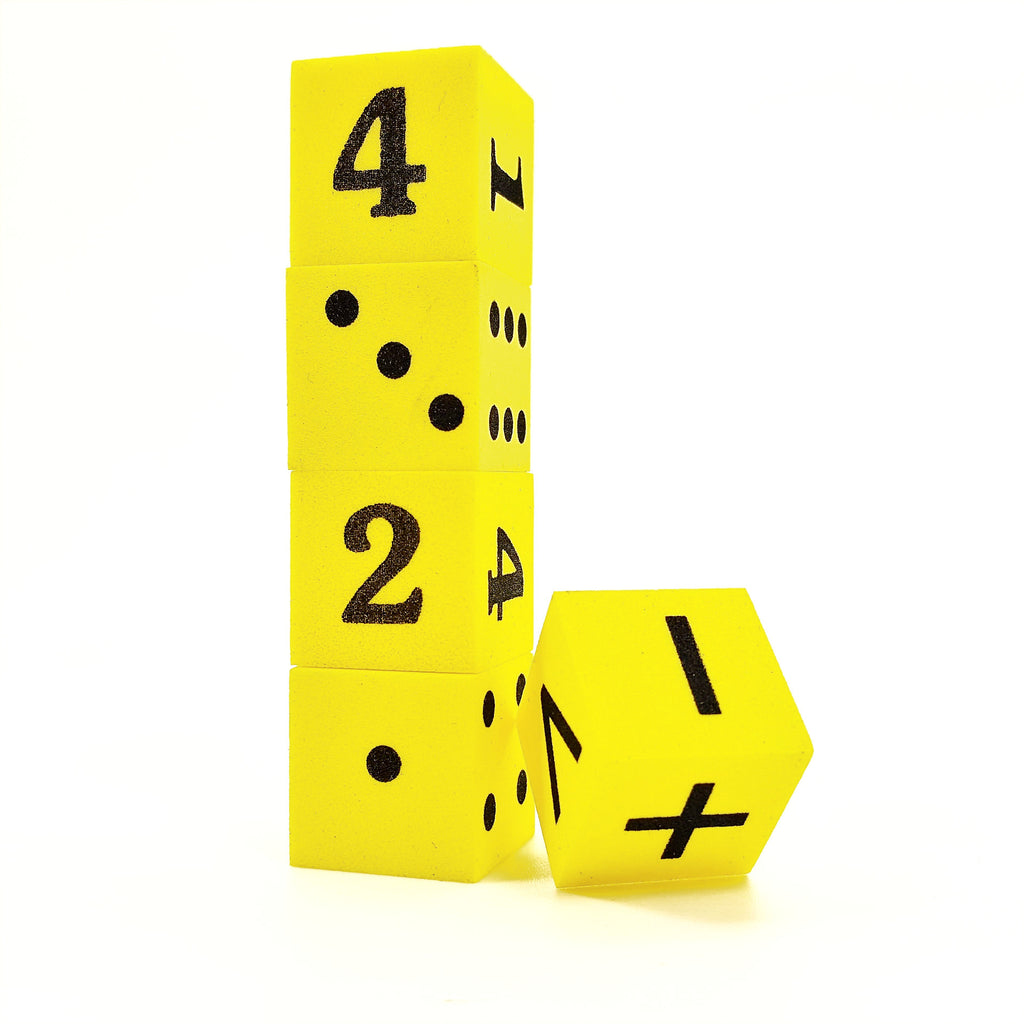•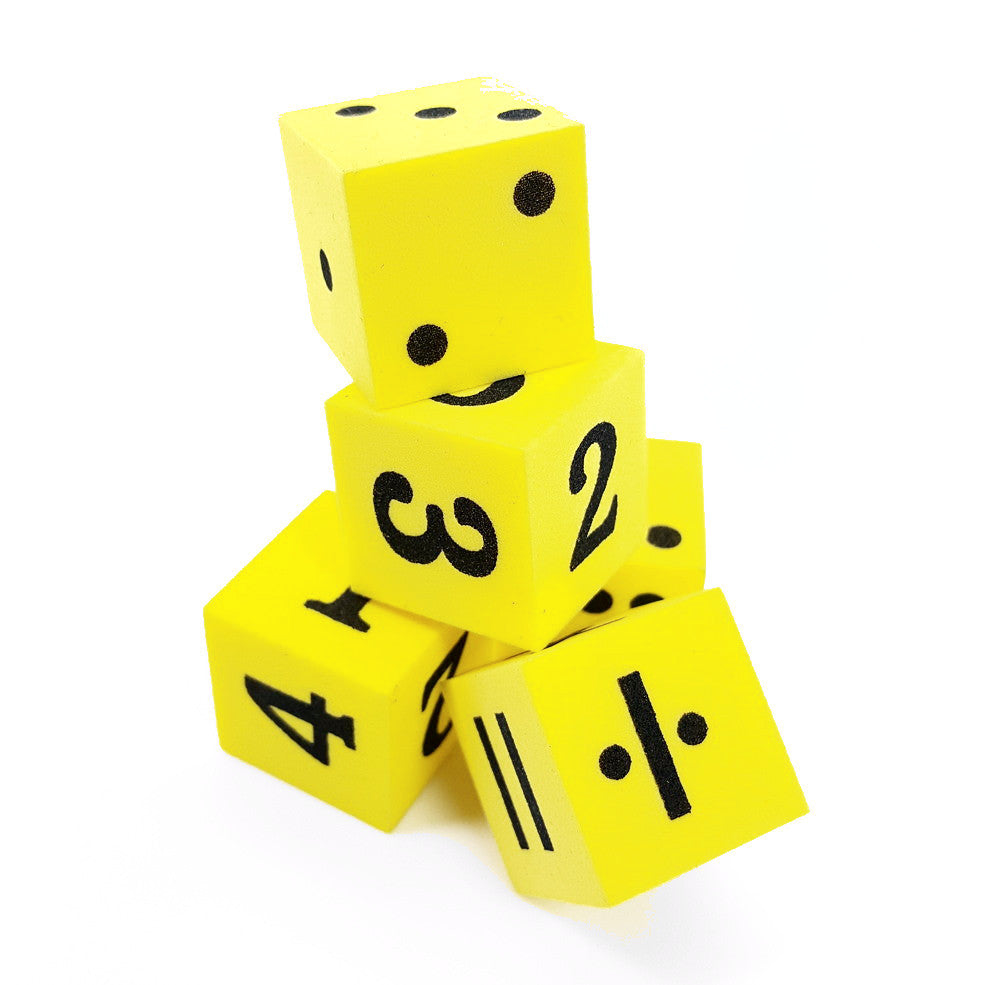# Mathematical Die - Mathematics Manipulative for Games, Number Recognition

• \$ 6.99
• Save \$ 2

• These Mathematical learning toys for 2-5 Year Old Boys & Girls is a great way to introduce kids to learn math in a fun way with gam.
• This math manipulative toy provides basic math concepts - Created to teach basic math arithmetics such as addition, subtraction, division, and multiplication.

## Mathematical Die Toys for Toddlers

A great set of math manipulatives for kids age group 1-5 to begin to discriminate and differentiate between numbers and dots on the dice.

Help kids learn by comparing and understanding that there are more than one way to show a particular number.

Time to practice your math skills by creating simple equations to complex ones using the math dice.

These game dice are great for reinforcing number recognition, playing classroom games and for teaching probability concepts. Learning has never been so much fun.

These hands on manipulatives make it easy and affordable to get children learning the hands-on way! Great for parents and as starter sets for teachers.

INCLUDES:

5 Dice (3/4 inch)

Sides include: standard dot, numbers and math symbols